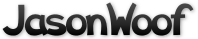Got questions, comments, patches, etc.? Contact Jason Woofenden
 author Joshua Grams Mon, 5 Mar 2007 19:47:21 +0000 (19:47 +0000) committer Joshua Grams Mon, 5 Mar 2007 19:47:21 +0000 (19:47 +0000)
 dust.c patch | blob | history dust.h patch | blob | history globals.h patch | blob | history main.c patch | blob | history rocks.c patch | blob | history rocks.h patch | blob | history score.c patch | blob | history score.h patch | blob | history sprite.c patch | blob | history sprite.h patch | blob | history

diff --git a/dust.c b/dust.c
index 4eeeb8b..ab58395 100644 (file)
--- a/dust.c
+++ b/dust.c
@@ -28,11 +28,11 @@ init_dust(void)
}

void
-move_dust(float ticks)
+move_dust(void)
{
int i;
-       float xscroll = screendx * ticks;
-       float yscroll = screendy * ticks;
+       float xscroll = screendx * t_frame;
+       float yscroll = screendy * t_frame;

for(i=0; i<N_DUST_MOTES; i++) {
motes[i].x -= xscroll / (1.3 + motes[i].z);
@@ -44,11 +44,11 @@ move_dust(float ticks)
}

void
-draw_dust(SDL_Surface *s)
+draw_dust(void)
{
int i;
-       uint16_t *pixels = s->pixels;
+       uint16_t *pixels = surf_screen->pixels;
for(i=0; i<N_DUST_MOTES; i++) {
-               pixels[s->pitch/2*(int)motes[i].y + (int)motes[i].x] = motes[i].color;
+               pixels[surf_screen->pitch/2*(int)motes[i].y + (int)motes[i].x] = motes[i].color;
}
}
diff --git a/dust.h b/dust.h
--- a/dust.h
+++ b/dust.h
@@ -11,7 +11,7 @@
#define MAX_DUST_DEPTH 2

void init_dust(void);
-void move_dust(float ticks);
-void draw_dust(SDL_Surface *);
+void move_dust(void);
+void draw_dust(void);

#endif // VOR_DUST_H
index c98f1f8..0b76cd7 100644 (file)
--- a/globals.h
+++ b/globals.h
@@ -19,6 +19,8 @@ extern SDL_Surface
*surf_rock[NROCKS],     // THE ROCKS
*surf_font_big; // The big font

+extern float t_frame;
+
extern font *g_font;

// Other global variables
diff --git a/main.c b/main.c
index 9e5c36f..c90a793 100644 (file)
--- a/main.c
+++ b/main.c
@@ -142,9 +142,9 @@ init_engine_dots() {

void
-new_engine_dots(float time_span) {
+new_engine_dots(void) {
int dir, i;
-       int n = time_span * ENGINE_DOTS_PER_TIC;
+       int n = t_frame * ENGINE_DOTS_PER_TIC;
float a, r;  // angle, random length
float dx, dy;
float hx, hy; // half ship width/height.
@@ -170,7 +170,7 @@ new_engine_dots(float time_span) {
dotptr->heat = 6;

// dot was created at a random time during the time span
-                               time = frnd() * time_span; // this is how long ago
+                               time = frnd() * t_frame; // this is how long ago

// calculate how fast the ship was going when this engine dot was
// created (as if it had a smooth acceleration). This is used in
@@ -185,7 +185,7 @@ new_engine_dots(float time_span) {

// the starting position (not speed) of the dot is calculated as
// though the ship were traveling at a constant speed for this
-                               // time_span.
+                               // t_frame.
dotptr->x = (ship.x - (ship.dx - screendx) * time) + s[dir]*hx;
dotptr->y = (ship.y - (ship.dy - screendy) * time) + s[(dir+1)&3]*hy;
if(dir&1) {
@@ -257,15 +257,15 @@ new_bang_dots(struct sprite *s)

void
-move_dot(struct dot *d, float ticks)
+move_dot(struct dot *d)
{
Sprite *hit;
float mass;

if(d->active) {
-               d->x += (d->dx - screendx) * ticks;
-               d->y += (d->dy - screendy) * ticks;
-               d->mass -= ticks * d->decay;
+               d->x += (d->dx - screendx) * t_frame;
+               d->y += (d->dy - screendy) * t_frame;
+               d->mass -= t_frame * d->decay;
if(d->mass < 0 || fclip(d->x, XSIZE) || fclip(d->y, YSIZE))
d->active = 0;
else {
@@ -281,38 +281,38 @@ move_dot(struct dot *d, float ticks)
}

void
-move_dots(float ticks)
+move_dots(void)
{
int i;

-       for(i=0; i<MAXBANGDOTS; i++) move_dot(&bdot[i], ticks);
-       for(i=0; i<MAXENGINEDOTS; i++) move_dot(&edot[i], ticks);
+       for(i=0; i<MAXBANGDOTS; i++) move_dot(&bdot[i]);
+       for(i=0; i<MAXENGINEDOTS; i++) move_dot(&edot[i]);
}

void
-draw_dot(SDL_Surface *s, struct dot *d)
+draw_dot(struct dot *d)
{
uint16_t *pixels, *pixel;
int row_inc;

if(d->active) {
-               pixels = (uint16_t *) s->pixels;
-               row_inc = s->pitch / sizeof(uint16_t);
+               pixels = (uint16_t *) surf_screen->pixels;
+               row_inc = surf_screen->pitch / sizeof(uint16_t);
pixel = pixels + (int)d->y * row_inc + (int)d->x;
*pixel = heatcolor[min(3*W-1, (int)(d->mass * d->heat))];
}
}

void
-draw_dots(SDL_Surface *s) {
+draw_dots(void) {
int i;

-       if(SDL_MUSTLOCK(s)) { SDL_LockSurface(s); }
-       draw_dust(s);
-       for(i=0; i<MAXBANGDOTS; i++) draw_dot(s, &bdot[i]);
-       for(i=0; i<MAXENGINEDOTS; i++) draw_dot(s, &edot[i]);
-       if(SDL_MUSTLOCK(s)) { SDL_UnlockSurface(s); }
+       if(SDL_MUSTLOCK(surf_screen)) { SDL_LockSurface(surf_screen); }
+       draw_dust();
+       for(i=0; i<MAXBANGDOTS; i++) draw_dot(&bdot[i]);
+       for(i=0; i<MAXENGINEDOTS; i++) draw_dot(&edot[i]);
+       if(SDL_MUSTLOCK(surf_screen)) { SDL_UnlockSurface(surf_screen); }
}

SDL_Surface *
@@ -503,7 +503,7 @@ void
draw(void)
{
SDL_FillRect(surf_screen,NULL,0);  // black background
-       draw_dots(surf_screen);            // background dots
+       draw_dots();            // background dots
draw_sprite(SPRITE(&ship));
draw_rocks();

@@ -519,7 +519,7 @@ draw(void)
// and fall through to
case HIGH_SCORE_DISPLAY:
// Display de list o high scores mon.
-                       display_scores(surf_screen, 150,50);
+                       display_scores(150,50);
break;
case GAMEPLAY:
@@ -576,9 +576,9 @@ init_score_entry(void)

// Count down the state timer, and change state when it gets to zero or less;
void
-update_state(float ticks)
+update_state(void)
{
-       state_timeout -= ticks*3;
+       state_timeout -= t_frame*3;
if(state_timeout > 0) return;

switch(state) {
@@ -673,7 +673,7 @@ gameloop() {
}

if(!paused) {
-                       update_state(t_frame);
+                       update_state();

// SCROLLING
tmp = (ship.y+ship.h/2 + ship.dy*t_frame - YSCROLLTO)/25 + (ship.dy-screendy);
@@ -687,12 +687,12 @@ gameloop() {

-                       move_sprites(t_frame);
-                       move_dots(t_frame);
-                       move_dust(t_frame);
+                       move_sprites();
+                       move_dots();
+                       move_dust();

-                       new_rocks(t_frame);
-                       new_engine_dots(t_frame);
+                       new_rocks();
+                       new_engine_dots();

collisions();

diff --git a/rocks.c b/rocks.c
index c404305..9384fe0 100644 (file)
--- a/rocks.c
+++ b/rocks.c
@@ -129,7 +129,7 @@ weighted_rnd_range(float min, float max) {
}

void
-new_rocks(float ticks)
+new_rocks(void)
{
int i, type;
struct rock *r;
@@ -138,7 +138,7 @@ new_rocks(float ticks)
float rmax;

if(nrocks < final_rocks) {
-               nrocks_timer += ticks;
+               nrocks_timer += t_frame;
if(nrocks_timer >= nrocks_inc_ticks) {
nrocks_timer -= nrocks_inc_ticks;
nrocks++;
@@ -148,7 +148,7 @@ new_rocks(float ticks)
rock_sides(ti, rmin, rmax);

// increment timers
-       for(i=0; i<4; i++) rtimers[i] += ti[i]*ticks;
+       for(i=0; i<4; i++) rtimers[i] += ti[i]*t_frame;

// generate rocks
for(i=0; i<4; i++) {
diff --git a/rocks.h b/rocks.h
index baa462d..1fbff53 100644 (file)
--- a/rocks.h
+++ b/rocks.h
@@ -3,7 +3,7 @@
void reset_rocks(void);

-void new_rocks(float ticks);
+void new_rocks(void);
void draw_rocks(void);

void blast_rocks(float x, float y, float radius);
diff --git a/score.c b/score.c
index 6823b57..b9c666e 100644 (file)
--- a/score.c
+++ b/score.c
@@ -157,7 +157,7 @@ show_score(void)
}

void
-display_scores(SDL_Surface *s, uint32_t x, uint32_t y)
+display_scores(uint32_t x, uint32_t y)
{
char t;
int i,h = font_height();
diff --git a/score.h b/score.h
index 6473260..2458135 100644 (file)
--- a/score.h
+++ b/score.h
@@ -37,7 +37,7 @@ void read_high_score_table(void);
void write_high_score_table(void);
int snprintscore(char *s, size_t n, int score);
void show_score(void);
-void display_scores(SDL_Surface *s, uint32_t x, uint32_t y);
+void display_scores(uint32_t x, uint32_t y);
int new_high_score(int score);
int insert_score(int score);
int process_score_input(SDL_keysym *key);
index 6a20442..3898123 100644 (file)
--- a/sprite.c
+++ b/sprite.c
@@ -128,11 +128,11 @@ reset_sprites(void)
}

void
-move_sprite(Sprite *s, float ticks)
+move_sprite(Sprite *s)
{
if(s->flags & MOVE) {
-               s->x += (s->dx - screendx)*ticks;
-               s->y += (s->dy - screendy)*ticks;
+               s->x += (s->dx - screendx)*t_frame;
+               s->y += (s->dy - screendy)*t_frame;
}
}

@@ -148,7 +148,7 @@ sort_sprite(Sprite *s)
}

void
-move_sprites(float ticks)
+move_sprites(void)
{
int sq;
@@ -158,7 +158,7 @@ move_sprites(float ticks)
-                       move_sprite(s, ticks); sort_sprite(s);
+                       move_sprite(s); sort_sprite(s);
}
}
set = 1-set;  // switch to other set of sprites.
index 41ab8f2..c95db83 100644 (file)
--- a/sprite.h
+++ b/sprite.h
@@ -41,8 +41,8 @@ void collisions(void);
void init_sprites(void);
void reset_sprites(void);